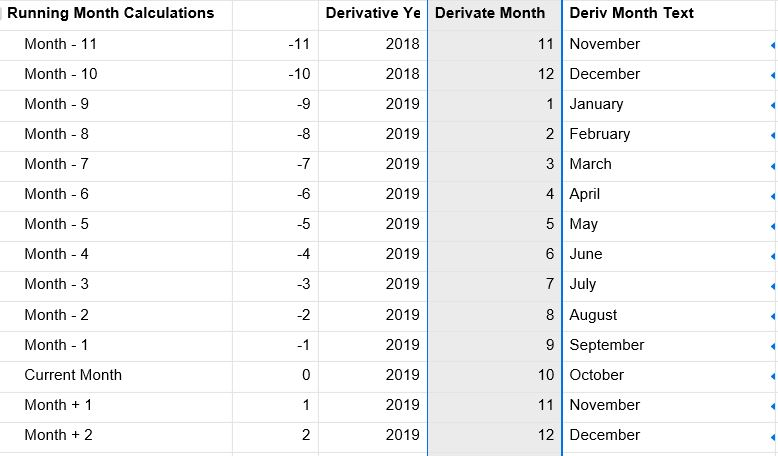# Using a modifier to derive month (formula de-bugging help

Ok. so I've set up a rolling dashboard built around the today function - something like this. Everything rolls over automatically as it all references TODAY() to determine the various derivatives.(see screenshot)

It's great, but doesn't scale (yet). The Month column uses this right now -

=IF((MONTH(TODAY()) + Modifier5) > 12, MONTH(TODAY()) + Modifier5 - 12, IF(MONTH(TODAY()) + Modifier5 <= 0, MONTH(TODAY()) + Modifier5 + 12, MONTH(TODAY()) + Modifier5))

Basically, if the derivative month goes over 12, subtract 12 so I can give use this column in a

DATE(year_reference, month_reference, day_reference) function for reporting further down.

I want to scale it for more than just the next (or last) year but keep running into errors, primarily around December - My current formula is this:

=INT(YEAR(TODAY()) + ((Modifier3 + MONTH(TODAY())) / 12)) to give me year

=MONTH(TODAY()) - ((DerivYear3 - YEAR(TODAY())) * 12 - Modifier3) to give me month

It works for any month except December. December returns the month as 0 and clicks year up a month early.

The problem is the underlined phrase. If the derived month is December (12), it equals a whole number and clicks the year over early. I'm trying to figure out an approach that will work.

Thank you.• Good Morning,
To get to month, I use a hidden column to assign the date code code of a cell by using "=MONTH(X)", and then I use an IF statement to define the number that is returned, "=IF(X = 1, "January", IF(X = 2, "February", IF(X = 3, "March", IF(X = 4, "April", IF(X = 5, "May", IF(X = 6, "June", IF(X = 7, "July", IF(X = 8, "August", IF(X = 9, "September", IF(X = 10, "October", IF(X = 11, "November", IF(X = 12, "December", "")))))))))))).

To get year, "=YEAR(X)
• I'm not trying to return a month name. I'm doing that using an index function and a separate date table. I'm trying to return what month & year it would be x months in the past or future, x being the modifier in my second column.

FYI - this is my month text function

=INDEX({Date Tables Range 3}, MATCH(DerivMonth5, {Date Tables Range 1}, 0))

This uses my derivative month number (integers 1 - 12) and returns the text name.

## Help Article Resources

Want to practice working with formulas directly in Smartsheet?

Check out the Formula Handbook template!